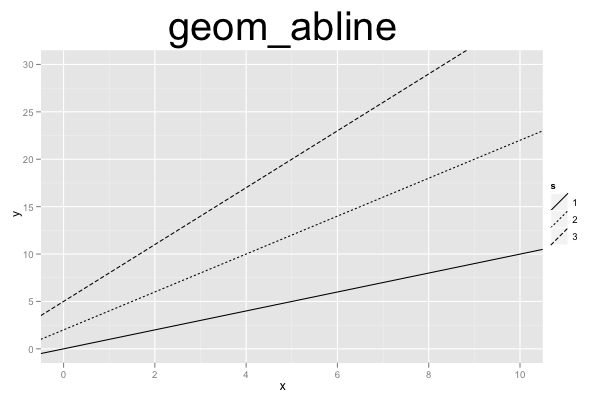## ggplot2 Quick Reference: geom_abline

A geom that draws a line defined by slope and y-axis intercept.

Default statistic: stat_abline

### Parameters

For geom_abline, whether or not one uses the default statistic (stat_abline) or the "do nothing" statistic (stat_identity), the available parameters and their meanings stay the same.

• slope - (required) slope of the line (the "a" in "y=ax+b")
• intercept - (required) intercept with the y axis of the line (the "b" in "y=ax+b")
• size - (default: 0.5) width of the line
• linetype - (default: 1=solid) the type of the line
• colour - (default: "black") the color of the line
• alpha - (default: 1=opaque) the transparency of the line

### Example

This example is slightly unrealistic, because the plot just contains an abline layer, without any other layers. Usually, `geom_abline()` is used to add a line to data shown in other layers (e.g. with `geom_point()`). Because there are no x or y aesthetics in this plot, ggplot2 cannot automatically produce useful x and y scales, and we have to explicitly define the scales to specify their limits (`scale_x_continuous(... limits=c(0,10))` and `scale_y_continuous(... limits=c(0,30))`). We use different line types for the three different lines. The linetype of each line is determined by the line's slope. Given that the linetype parameter expects a discrete variable, we convert the continuous data\$s into a discrete factor (with `factor(s)`). Because ggplot2 would use "factor(s)" as the default name of the legend, we use `scale_linetype(name="s")` to set the legend's title to the more intuitive "s".```d=data.frame(s=c(1,2,3), ic=c(0,2,5))
ggplot() +
scale_x_continuous(name="x", limits=c(0,10)) +
scale_y_continuous(name="y", limits=c(0,30)) +
scale_linetype(name="s") +
geom_abline(data=d, mapping=aes(slope=s, intercept=ic, linetype=factor(s))) +
opts(title="geom_abline", plot.title=theme_text(size=40, vjust=1.5))```